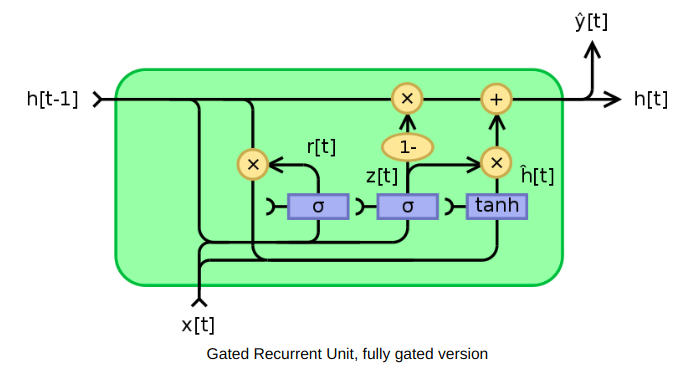# Some common Machine Learning, Statistics and Data Science terms starts with G

## G

[su_table]

### Word ### Description
Gated Recurrent Unit (GRU) The GRU is a variant of the LSTM (Long Short Term Memory) and was introduced by K. Cho. It retains the LSTM’s resistance to the vanishing gradient problem, but because of its simpler internal structure it is faster to train.

Instead of the input, forget, and output gates in the LSTM cell, the GRU cell has only two gates, an update gate z , and a reset gate r . The update gate defines how much previous memory to keep, and the reset gate defines how to combine the new input with the previous memory.|
|Ggplot2|GGplot2 is a data visualization package for the R programming language. It is a highly versatile and user-friendly tool for creating attractive plots.

It can be easily installed using the following code from the R console:

install.packages(“ggplot2”)|
|Go|Go is an open source programming language that makes it easy to build simple, reliable, and efficient software. Go is a statically typed language in the tradition of C.

The main features of Go are:

• Memory safety
• Garbage collection
• Structural typing

The compiler and other tools originally developed by Google are all free and open source.

|Goodness of Fit|The goodness of fit of a model describes how well it fits a set of observations. Measures of goodness of fit typically summarize the discrepancy between observed values and the values expected under the model.

With regard to a machine learning algorithm, a good fit is when the error for the model on the training data as well as the test data is minimum. Over time, as the algorithm learns, the error for the model on the training data goes down and so does the error on the test dataset. If we train for too long, the performance on the training dataset may continue to decrease because the model is overfitting and learning the irrelevant detail and noise in the training dataset. At the same time the error for the test set starts to rise again as the model’s ability to generalize decreases.

So the point just before the error on the test dataset starts to increase where the model has good skill on both the training dataset and the unseen test dataset is known as the good fit of the model.|
|Gradient Descent|Gradient descent is a first-order iterative optimization algorithm for finding the minimum of a function. In machine learning algorithms, we use gradient descent to minimize the cost function. It find out the best set of parameters for our algorithm. Gradient Descent can be classified as follows:

• On the basis of data ingestion:
1. Full Batch Gradient Descent Algorithm
2. Stochastic Gradient Descent Algorithm

In full batch gradient descent algorithms, we use whole data at once to compute the gradient, whereas in stochastic we take a sample while computing the gradient.

• On the basis of differentiation techniques:
1. First order Differentiation
2. Second order Differentiation|